Notes for September 23 class -- Reflection, Refraction, Polyhedra

STRUCTS

GLSL supports structs, which makes it much easier to organize your program and pass data around. For example, you might describe the material properties needed for Phong shading as follows:

```   struct Material {
vec3  ambient;
vec3  diffuse;
vec3  specular;
float power;
};
```
This lets you store various materials as an array of structs:
```   uniform Material uMaterials[NS];
```
By storing this data as a uniform variable, we make it possible to pass the data down from the CPU to the GPU, which we do as described in the next section.

You might want to do the same with your spheres. Rather than just use a vec4, you can create a struct for shapes. That way you will also be able to accommodate shapes that are not spheres.

For example, you might want to implement something like:

```   struct Shape {
int   type;   // 0 for sphere. 1,2,etc for other kinds of shapes.
vec3  center;
float size;
};
```
and declare an array of shapes:
```   uniform Shape uShapes[NS];
```

SENDING DATA FROM THE CPU TO THE GPU

There are two parts to passing data from the CPU to the GPU:

1. Storing the location of a uniform variable in the shader (needed only once)
2. Setting the value for that variable (which can be done every animation frame)
You will be doing both of these steps in the Javascript file worlds/week3.js. In that file, look for the following line:
```   state.uTimeLoc = gl.getUniformLocation(program, 'uTime');
```
That is the line where we store the location of the uniform shader variable 'uTime'. Note that function gl.uniformLocation() takes two arguments: The compiled shader program and the name of the variable.

The value it returns is simply an offset, in bytes, into the compiled shader program that is running on the GPU.

You can add similar declarations into the code right after that line. For example, to store the locations of all of the members of the first material in the uMaterials array, you might write:

```   state.uMaterialsLoc = [];
state.uMaterialsLoc = {};
state.uMaterialsLoc.diffuse  = gl.getUniformLocation(program, 'uMaterials.diffuse');
state.uMaterialsLoc.ambient  = gl.getUniformLocation(program, 'uMaterials.ambient');
state.uMaterialsLoc.specular = gl.getUniformLocation(program, 'uMaterials.specular');
state.uMaterialsLoc.power    = gl.getUniformLocation(program, 'uMaterials.power');
```
Note that you need to do this separately for each member of each element in an array of structs in your shader program.

The second step of the process, which generally happens once per animation frame, is to set the value of your uniform variables. In worlds/week3.js look for a line that starts:

```    gl.uniform1f(state.uTimeLoc,
```
This is where the actual value for uniform variable 'uTime' is set at each animation frame.

To set the values for uniform shader variable uMaterials, you might, for example, follow the above line of code with the following sequence of lines:

```    gl.uniform3fv(state.uMaterialsLoc.ambient , [.05,0,0]);
gl.uniform3fv(state.uMaterialsLoc.diffuse , [.5,0,0]);
gl.uniform3fv(state.uMaterialsLoc.specular, [.5,.5,.5]);
gl.uniform1f (state.uMaterialsLoc.power   , 20);
```
There are several things to note about the above. For one thing, there are various related methods to set the value of a uniform variable, depending on the type of that variable. In the above example we see two such methods:
1. gl.uniform3fv() // set the value of a uniform of type vec3
2. gl.uniform1f () // set the value of a uniform of type float
The 'v' at the end of 'uniform3fv' indicates that we specify the value via a single array argument. We could also have set the components of any vec3 individually by calling, for example:
```    gl.uniform3f(state.uMaterialsLoc.ambient, .05, 0, 0);
```

MIRROR REFLECTION

 After doing Phong shading, we might add mirror reflection. To do this, we need to cast a secondary ray from the surface of our object and see whether that secondary ray hits any other objects. If the secondary ray manages to hit any other objects, then we perform Phong shading at the nearest such object along the secondary ray, and mix that color in. The basic algorithm is as follows: Form a secondary "mirror reflection" ray Send that ray out from the surface and see what it hits If it hits any object, Phong shade the nearest such object Add in the result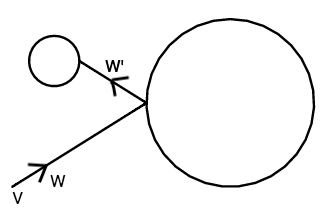To form the secondary ray, we use the incoming ray direction W and the surface normal N to compute a mirror reflection ray W', using essentially the same technique you used last week to calculate a mirror reflection for our light vector L:

W' = W - 2 (N ● W) N
It's not exactly the same, because W points toward the surface, unlike L which pointed away from the surface, so the above equation is actually the negative of the one we used to calculate R.

We can now shoot a ray into the scene and see whether it hits any objects. If so, we will be shading the nearest such object.

To compute a mirror surface reflection for shape uShapes[i] with material uMaterials[i] at surface point P (with surface normal N), the algorithm looks roughly like this:

```   if (length(uMaterials[i].reflect) > 0.)     // if reflection color is any
// color other than black
W' = W - 2 (N ● W) N
tMin = 1000
Shape S
Material M
vec3 P', N'
for (int j = 0 ; j < NS ; j++)
t = rayShape(P, W', uShapes[j]).x     // use only first of the two roots
if t > 0 and t < tMin
S = uShapes[j]
M = uMaterials[j]
P' = P + t * W'                    // find point on surface of other shape
N' = computeSurfaceNormal(P', S)   // find surface normal at other shape
tMin = t
if (tMin < 1000)
rgb = phongShading(P', N', S, M)      // do phong shading at other shape
color += rgb * uMaterials[i].reflect  // tint and add to color
```
Notice from the above that it could be useful to add the following member to your Material struct:
```   struct Material {
...
vec3 reflect; // Reflection color. Black means no reflection.
...
};
```

REFRACTION

Refraction is a little trickier than reflection because you need to use Snell's law to trace the ray through your shape, and then trace a ray from there out into the scene to see whether any other objects are visible due to refraction through your transparent shape.

Starting with a ray in direction W arriving at surface point P with normal N, we first need to compute the new ray direction W' into the shape due to refraction.

Then we need to form a new ray from slightly outside the surface through the transparent shape, to find the exit point at the rear of the shape. To do this, we will need to use the second root of the ray shape computation (up until now, we have used only the first root).

Then we need to use Snell's law a second time change the ray direction once again, because the ray will again be refracted as it emerges from the rear of the transparent shape back into the air.

Finally, we need to see what object (if any) the refracted ray hits, do a Phong computation at that other shape, and add in the result, tinted by the color of our refractive material. Note that this last step is very similar to what we do for reflections.

Computing a refracted ray:

In order to refract a ray using Snell's law, we need to bend from W to W'. Suppose the surface normal is N.

If θ1 is the angle between the incoming ray W and -N, and θ2 is the angle between the emergent ray W' and -N, then Snell's law tells us that:

sin(θ2) = sin(θ1) / index_of_refraction

To compute W', we first decompose W into two orthogonal vectors Wc which is parallel to N, and Ws which is orthogonal to N:

Wc = (W ● N) N

Ws = W - Wc

Then we can use Ws and Snell's law to compute W's, the component of the emergent ray which is orthogonal to surface normal N:
W's = Ws / index_of_refraction
From there it's easy to compute the component of the emergent ray W' which is parallel to N, and that gives us the answer:
W'c = -N * sqrt(1 - W's ● W's)

W' = W'c + W's

The algorithm then looks roughly like this:

```    if (length(uMaterials[i].transparent) > 0.)        // If transparent color is not black

// Compute ray that refracts into the shape

W' = refractRay(W, N, uMaterials[i].indexOfRefraction)
t' = rayShape(P - W'/1000, W', uShapes[i]).y    // Note: We are using the second root,
//      where the ray exits the shape.
// Compute second refracted ray that emerges back out of the shape

P' = P + t' * W'
N' = computeSurfaceNormal(P', uShapes[i])
W" = refractRay(W', N', 1 / uMaterials[j].indexOfRefraction)

// If emergent ray hits any shapes, do Phong shading on nearest one and add to color

Shape S
Material M
tMin = 1000
vec3 P", N"
for (int j = 0 ; j < NS ; j++)
t = rayShape(P', W", uShapes[j]).x
if t > .001 && t < tMin
S = uShapes[j]
M = uMaterials[j]
P" = P' + t * W"                         // find point on surface of other shape
N" = computeSurfaceNormal(P", S)         // find surface normal at other shape
tMin = t;
if tMin < 1000
rgb = phongShading(P", N", S, M)            // do phong shading at other shape
color += rgb * uMaterials[i].transparent    // add in tinted result
```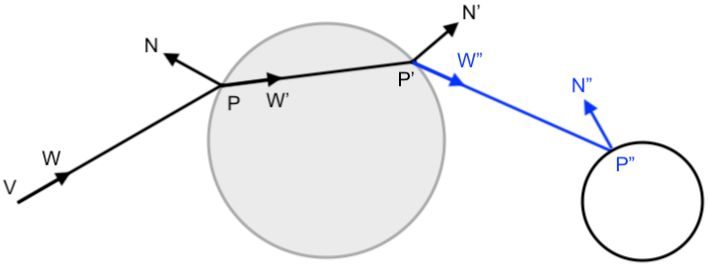Notice from the above that it could be useful to add the following members to your Material struct:

```   struct Material {
...
vec3 transparent;        // Transparency color. Black means the object is opaque.
float indexOfRefraction; // Higher value means light will bend more as it refracts.
...
};
```

RAY TRACING TO A HALF SPACE

Before we can ray trace to a polyhedron (like a cube or pyramid or octahedron), first we need to know how to ray trace to a single half space. Then we will be able to ray trace to polyhedral shapes, because such shapes are intersections of half spaces.

A halfspace is bounded by a plane P = (a,b,c,d). In order to properly think about the interaction between points and planes, we need to be a little more precise in our math.

A short detour into homogeneous coordinates:

A point in space V is properly written as a column vector:

 V = xyzw
The w above is the homogeneous coordinate. We generally normalize a point by dividing through by its homogeneous coordinate. The homogeneous coordinate then equals 1.

If w is zero, then we are dealing with a point at infinity, also known as a direction vector.

Using this more precise way of writing things, the parametric ray tracing equation V + tW, which contains point V and direction vector W, is more properly written as:

 Vx Vy Vz 1 +   t Wx Wy Wz 0
Notice that when this is written as a single column vector, the homogeneous coordinate remains 1, no matter what the value of t:
 Vx + t Wx Vy + t Wy Vz + t Wz 1

Interaction between a point and a halfspace:

Since any plane P is a row vector, and any point V is a column vector, multiplying them is basically performing a dot product, with the result being a single numerical value:

 P ● V   =   Pa Vx + Pb Vy + Pc Vz + Pd Vw   =   ( Pa Pb Pc Pd ) Vx Vy Vz Vw
We say that a point is on a plane when that numerical value is zero.

Now we can precisely define a halfspace P as the set of all points V in space such that: P ● V ≤ 0

A point can be inside, outside or on the surface of a halfspace:

• Any point for which P ● V < 0 is strictly inside the halfspace.

• Any point for which P ● V > 0 is outside the halfspace.

• Any point on the bounding plane P ● V = 0 is on the surface of the halfspace.

Ray tracing to a halfspace:

Given a ray V + t W and a halfspace defined as all points V such that P ● V ≤ 0, we can find the intersection of the ray with the bounding plane of the halfspace by finding the value of t for which:

P ● (V + t W) = 0
We can rewrite this as (P ● V) + t (P ● W) = 0.

Remember that V is a point and W is a direction vector, so the values we will use for V and W in the two above dot products are, respectively:

 Vx Vy Vz 1 and Wx Wy Wz 0

Solving for t, we get:

 t   = - (P ● V)   (P ● W)
There are four cases to consider:
• If the ray origin is outside the halfspace (that is, P ● V > 0), then:

• Case 1: If t < 0, then the ray has missed the halfspace.

• Case 2: If t > 0, then the ray is entering the halfspace at point V + tW.

• If the ray origin is inside the halfspace (that is, P ● V < 0), then:

• Case 3: If t > 0, then the ray is exiting the halfspace at point V + tW.

• Case 4: If t < 0, then the entire ray is contained within the halfspace.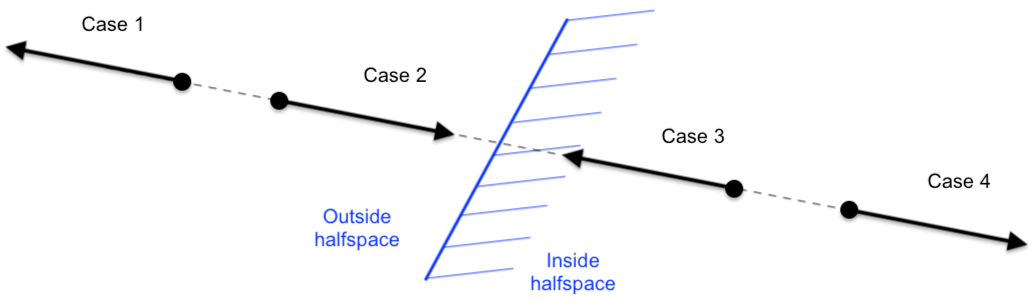RAY TRACING TO A POLYHEDRON

A polyhedron can be thought of as an intersection of halfspaces. For example, a cube is an intersection of six halfspaces, and an octahedron is an intersection of eight halfspaces.

Ray tracing to a single halfspace results in a range of values for t. Ray tracing to a polyhedron consists of taking the intersection of all of those ranges.

In practice, this means starting with a very large range for t, such as [tmin, tmax] = [-1000, 1000]). Then we loop through each of the defining halfspaces [a,b,c,d]i for that polyhedron, and possiblity whittling down this range at each step.

If the range is non-empty after we have finished this process, then the ray has intersected the polyhedron.

For any given face of the polyhedron, we consider each of the four cases of a ray intersecting a halfspace.

• If we encounter Case 1, then we know the ray has missed the polyhedron.

• If we encounter Case 2:
if t > tmin
frontSurfaceNormal = [a,b,c]i
tmin = t

• If we encounter Case 3:
if t < tmax
rearSurfaceNormal = [a,b,c]i
tmax = t

• If we encounter Case 4, then we do nothing.
At the end of this process, if we never encountered Case 1, and tmin ≤ tmax, then the ray intersects the polyhedron in the range tmin ≤ t ≤ tmax. Otherwise, the ray misses the polyhedron.

Some useful polyhedra:

Consider a halfspace as defined by ax + by + cd + d ≤ 0. Then the six planes that define a cube centered at the origin of size 2r are:

 ``` a b c d ----------- -1 0 0 -r 1 0 0 -r 0 -1 0 -r 0 1 0 -r 0 0 -1 -r 0 0 1 -r ```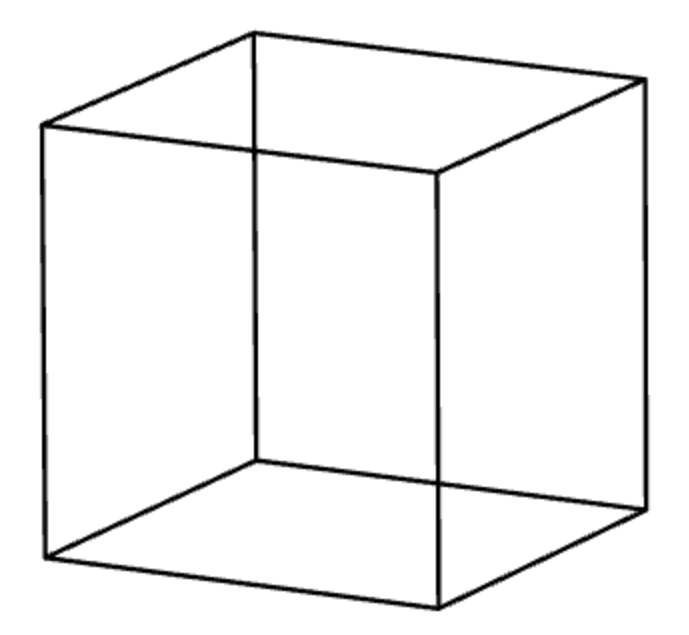The eight planes that define an octahedron centered at the origin of size 2r are:
 ``` a b c d -------------- -r3 -r3 -r3 -r r3 -r3 -r3 -r -r3 r3 -r3 -r r3 r3 -r3 -r -r3 -r3 r3 -r r3 -r3 r3 -r -r3 r3 r3 -r r3 r3 r3 -r ```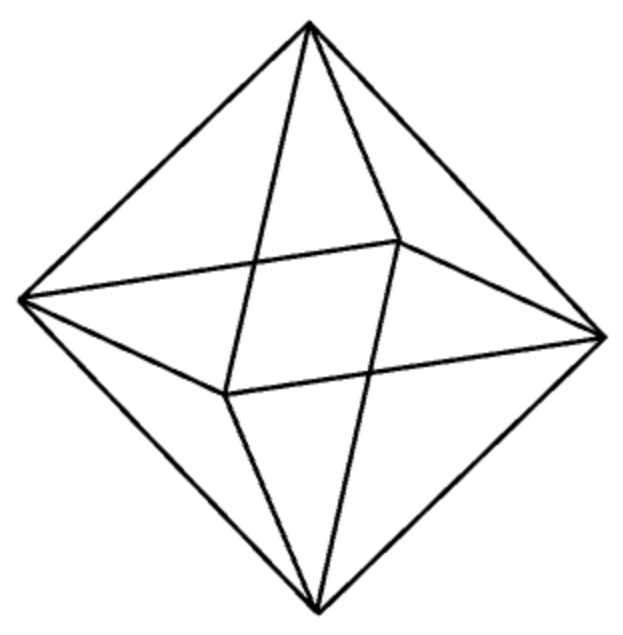where r3 is 1 / sqrt(3), so that the surface normal will be of unit length.

To ray trace to a polyhedron with an arbitrary center point C, we can first modify our ray by replacing V with V-C, then ray trace using our modified ray to the polyhedron defined at the origin.

ABSTRACTING OUT THE SHAPE

You might want to consider creating a single wrapper function called rayShape() that works for spheres and different kinds of polyhedra, internally splitting the logic of tracing a ray into different cases, based on the type of shape. In all cases, your rayShape function would return a vec2 to indicate the values of t where the ray enters and exits the shape, respectively.

This will allow you to use your logic for such actions as shadows, reflection and refraction in a scene that contains a mix of different kinds of shapes.

Similarly, you might want to consider creating a single wrapper function called computeSurfaceNormal(), which takes a point and a shape as arguments.

A tip on implementation:

In the case of ray tracing to a polyhedron, you can actually do the logic of computing the entering and exiting surface normals when you ray trace to the polyhedron, save these results to variables, and then use those stored values when computeSurfaceNormal() is called.

This works because computeSurfaceNormal() is always called right after the corresponding call to rayShape().

HOMEWORK

For your homework, which is due by the start of class on Monday Sept 30, implement reflection, refraction and ray tracing to polyhedra.

By the way, you can use procedural textures that include the noise() function in very interesting ways for ray tracing. In addition to textures, you can also use it to vary the direction of the ray at various stages of your rendering process, to create very interesting shading and reflection/refraction effects. You might want to try playing with that.

We have an improved version of the library package as hw3.zip, which has better error reporting. Or you can just make a copy of the library package from last week.

All of your changes are going to be confined to just two files: